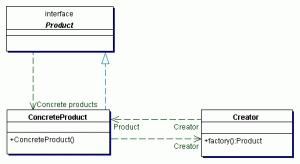# 设计模式学习总结-简单工厂方法模式public interface Operation {   public double getResult(double a, double b);   }   public class OperationAdd implements Operation{   public double getResult(double a, double b){ return a + b; } }   public class OperationDiv implements Operation{   public double getResult(double a, double b){ return a / b; } }   public class OperationMul implements Operation{   public double getResult(double a, double b){ return a * b; } }   public class OperationSub implements Operation{   public double getResult(double a, double b){ return a - b; } }
public class SimpleFactory {   public static Operation createOperation(char operate){ Operation oper = null; switch (operate){ case '+': oper = new OperationAdd(); break; case '-': oper = new OperationSub(); break; case '*': oper = new OperationMul(); break; case '/': oper = new OperationDiv(); break; } return oper; } }
 public static void main(String[] args){ double a = 8; double b = 2; Operation add = SimpleFactory.createOperation('/'); double result = add.getResult(a,b); System.out.println(result); }

## 6 Comments on this Post.

1.一直在看你这个系列 不错噢 学习了 ^_^

2.呵呵 你也认识Jackal啊 BYR? 幸会幸会

3.只是也订阅了他的博客 不是咯 uestc O(∩_∩)O~
ps:你博客不能引用回复啊？

4.5.